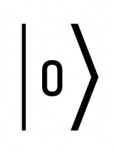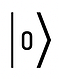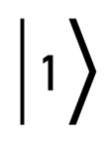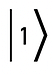# Introduction to Quantum Computing

Interior of IBM Quantum computing system. (Credit: IBM)

A video presentation of the blog post:

Quantum computers are vastly different from classical digital computers. The first question you might ask is, “what is a quantum computer?”, and the second, “why do we need them?” Joe Moreno, Director of Development at CANA Advisors, answers these questions in his complex, yet accessible (and fascinating) post on quantum computers.

The fundamentals of quantum mechanics go back to the end of the 19th century with theoretical physicist Max Planck. In the intervening years, minds such as Albert Einstein, Niels Bohr, Werner Heisenberg, and Erwin Schrödinger contributed to the quantum field. The idea of using quantum particles to store and process information was proposed by physicist Richard Feynman in 1981, and now companies such as IBM operate dozens of actual quantum computing systems. The quantum computing field has not yet scratched the surface of what is possible, but the groundwork has been laid. Let’s take a look by discovering the differences between classical digital computers and quantum computers.

### Processing

Digital computers process data in series. Data is processed, sequentially, through a central processing unit (CPU). Even in a computer with multiple CPUs, they still need to explicitly coordinate operations.

Quantum computers process data in parallel. This is how the universe actually works. There is no single central place in the universe that processes the laws of physics. If we think of our body as a computer, our brain would be the processor. Yet our brain doesn’t need to explicitly coordinate many of our body’s activities. For example, if I take a bite out of an apple, swallow it, and then drop dead (resulting in no more brain activity), the apple would continue to digest in my stomach, at least for a little while, because that activity happens in parallel with other bodily functions.

### Performance

Digital computer performance increases by a factor of two for each bit added (2n). A computer that can process eight bits is twice as powerful as a computer that can process seven bits.

Quantum computer performance increases exponentially for each bit added (2ⁿ) due to its parallel nature. This means a quantum computer that can process 64 bits can be thought of as 2⁶⁴ (a 20 digit number) in terms of how much information it can store and process.

### Behavior

Digital computers use simulation to solve problems. We write computer programs that store variables and execute algorithms to simulate what we observe in the real world. For example, if we want to simulate the interaction between the coronavirus and a candidate COVID-19 vaccine, we’d take the parameters that describe both, assign them to variables in a computer program, and write algorithms to simulate the interactions. The better our algorithm, the better our results. However, this only works on a small scale because there are too many variables and interactions to consider on the scale of a human.

Quantum computers use imitation to solve problems. A good example of this is a lab rat. Before we give a candidate vaccine to a human, we test it on a lab rat. In this scenario, we can think of the lab rat as a computer that is imitating how the human body works.

### Information

Digital computers store information in classical bits. A bit can only be a zero or one. Keep in mind that computer science, much like political science, is a human-created science, so we have defined the conditions and rules. Computer scientists have defined a bit to explicitly be a zero or a one.

Quantum computers store information in quantum bits (qubits). A qubit can be a zero or one or somewhere in between zero and one, or negative, or a complex number all at the same time. To wrap your head around how a qubit can be in many different states at the same time, consider using a coin to represent state. Let’s say heads is one and tails is zero. What state is the coin in when we flip it and it’s spinning through the air? Is it heads? Is it tails? Is it both? Is it neither?

A qubit is represented as a ket (vector) which distinguishes them from classical bits.

A zero qubit is represented as:A one qubit is represented as:### Logic

Digital computers perform operations using logic gates governed by Boolean algebra (AND, OR, NOT, XOR, etc.). By performing operations on multiple bits, at the same time, we can produce meaningful information.

Quantum computers perform operations using quantum logic gates (X, Y, Z, CNOT, Hadamard, etc.) governed by linear algebra (matrix algebra). These quantum logic gates are somewhat different from digital logic gates. For example, the Hadamard gate is used to quantumly entangle subatomic particles in a quantum computer and then put the particles into a state of superposition to process information. Borrowing from my earlier example of flipping a coin, we can think of superposition, in an overly simplified way, as when the coin is flipping through the air.

### Storage

Digital computers store bits using voltage and charge. This is done inside “chips” (integrated circuits) made up mostly of transistors. A transistor is simply a switch that can be turned on (one) and off (zero) without moving atoms – transistors only move electrons.

Quantum computers store information in qubits using particle spin. Quantum particles have different properties such as mass, charge, and spin. When a particle is in a state of superposition we can think of it as spinning, much like flipping a coin. When we measure (read) the coin’s state after flipping it, it has only one of two outcomes, heads or tails. Similarly, quantum particles end up in only one of two states when we measure them called spin up or spin down . We can think of spin up as when its axis is pointing up (north), which we call zero, and we can think of spin down as when its axis is pointing down (south), which we call one.

### Architecture

Digital computers use a von Neumann model with a CPU, ALU (arithmetic logic unit), and memory to store instructions and data. Physically, bits are stored in transistors at room temperature.

Quantum computers store and process data using quantum error correction (this is what makes a quantum computer a quantum computer) . Quantum error correction protects the qubits (electrons, photons, nuclei, etc.) from outside interference. The biggest source of interference is heat. Physically, qubits are stored at less than 1 Kelvin (about 0.015 Kelvin) to remove any thermal noise that could disturb them. The requirement to maintain a temperature of less than -457.87 degrees Fahrenheit presents its own set of operational challenges.

### Output

Digital computers are deterministic. The exact same inputs, into the exact same algorithms, always yields the exact same outputs.

Quantum computers are probabilistic. Repetition of the same inputs yields probabilistic outputs. This is a realistic model of how the universe works. If we flip a coin 10,000 times, we’d expect the sum of heads and the sum of tails to total 10,000 with about a 50/50 split. However, it’s entirely possible that the coin could land on its side (edge). Quantum computers can better represent real-world probabilities.

### Reversibility

Digital computers’ logic gates are not all reversible. If I show you the outputs from a digital computer, and tell you which operation is performed that yielded those outputs, you may still not have enough information to figure out the inputs.

Quantum computers’ logic gates are all reversible. If I show you the outputs from an operation, and tell you the operation that was performed, you could always determine all of the inputs. So much so that if you took these outputs and put them through the same operation, as inputs, your new outputs would match the original inputs in the first step.

### Current State of Quantum Computing

Before 2020, researchers viewed quantum computing as primarily a scientific endeavor with relatively little immediate bearing on the commercial viability of quantum computing. However, that has quickly changed with multiple companies entering the quantum computing market. Amazon currently has a web service where anyone can run quantum computing tasks on a variety of quantum computers. The ability to decode complex cryptographic challenges, process enormous amounts of data quickly, and simulate atomic and subatomic behavior in a controlled environment is likely just the tip of the quantum wave to come.